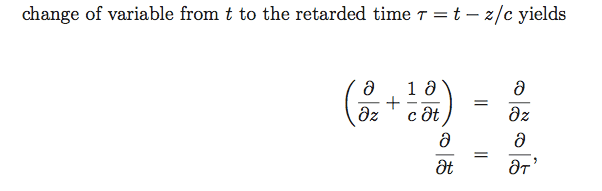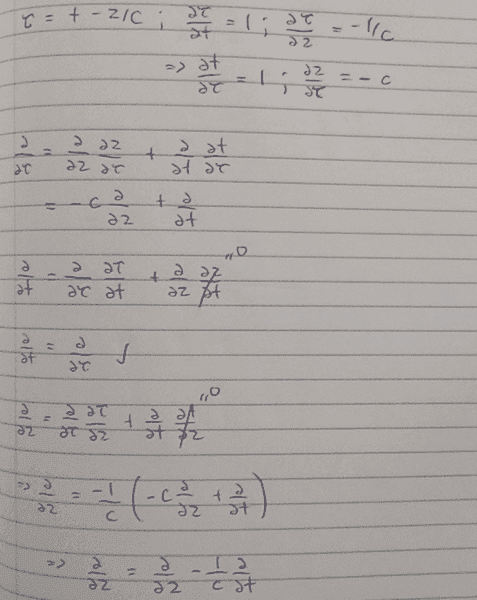# Change of variable - partial derivative

• II am trying to prove that the above is true when performing the change of variable shown. Here is my attempt:What I am not quite understanding is why they choose to isolate the partial derivative of ##z## on the right side (as opposed to the left) that I have in my last line. This ultimately amounts to switching the sign of ## \frac{1}{c} \frac {\partial}{\partial t} ## but what differentiates the ## \frac {\partial}{\partial z} ## on the left and right sides? Why is it correct to switch terms over and isolate the partial derivative of ##z## that I have in my solution's right side? It seems like they just add ## \frac{1}{c} \frac {\partial}{\partial t} ## but this has slightly confused me as to why ## \frac {\partial}{\partial z} ## appears invariant upon exchange of ## \pm \frac{1}{c} \frac {\partial}{\partial t} ##. Any explanations for what I am certainly missing would be greatly appreciated.

Last edited:

andrewkirk
Homework Helper
Gold Member
Where did these formulas come from?
The first formula doesn't look correct because it implies that ##\frac\partial{\partial t}## is zero.

Where did these formulas come from?
The first formula doesn't look correct because it implies that ##\frac\partial{\partial t}## is zero.

The one with the pure white background is from a paper. It is from B1 in the appendix: http://arxiv.org/pdf/1601.01717v2.pdf

I believe it is stating that the entire operator contained in the parentheses is equivalent to the partial derivative of ##z## with the change of variable. I've done the math above and I get similar results (well if I switch ##\frac{1}{c} \frac\partial{\partial t}## to the other side). But I agree, I don't quite see how it makes sense at a first glance at least intuitively.

andrewkirk
Homework Helper
Gold Member
I would put it down to careless writing on the authors' part. I expect they have something in mind that makes sense, but they have made mistakes in translating that into mathematical notation and ended up with something that makes no sense.

If you are familiar with the subject matter of the paper you may be able to guess what it is that they were trying to say. Alternatively, you could contact the authors and ask them what they meant.

One other comment: The manuscript appears to be using a version of the Total Derivative formula, that if ##y## is a function of ##t## and ##x_1,...,x_n##, and each of the ##x_k## is a function of ##t##, then:
$$\frac{dy}{dt}=\frac{\partial y}{\partial t}+\sum_{k=1}^n\frac{\partial y}{\partial x_k}\frac{dx_k}{dt}$$
However in the manuscript, the total derivatives (items using ##d## instead of ##\partial##) are replaced by partials. That is not necessarily valid or even well-defined and can lead to circularity or ambiguity.

I would put it down to careless writing on the authors' part. I expect they have something in mind that makes sense, but they have made mistakes in translating that into mathematical notation and ended up with something that makes no sense.

If you are familiar with the subject matter of the paper you may be able to guess what it is that they were trying to say. Alternatively, you could contact the authors and ask them what they meant.

One other comment: The manuscript appears to be using a version of the Total Derivative formula, that if ##y## is a function of ##t## and ##x_1,...,x_n##, and each of the ##x_k## is a function of ##t##, then:
$$\frac{dy}{dt}=\frac{\partial y}{\partial t}+\sum_{k=1}^n\frac{\partial y}{\partial x_k}\frac{dx_k}{dt}$$
However in the manuscript, the total derivatives (items using ##d## instead of ##\partial##) are replaced by partials. That is not necessarily valid or even well-defined and can lead to circularity or ambiguity.

Thank you for pointing that out. To my knowledge, they are trying to take the partial derivative and the reasoning for going from the partial to total derivative is not obvious to me. I do know the authors and certainly will contact them to clarify this point.

I would put it down to careless writing on the authors' part. I expect they have something in mind that makes sense, but they have made mistakes in translating that into mathematical notation and ended up with something that makes no sense.

If you are familiar with the subject matter of the paper you may be able to guess what it is that they were trying to say. Alternatively, you could contact the authors and ask them what they meant.

One other comment: The manuscript appears to be using a version of the Total Derivative formula, that if ##y## is a function of ##t## and ##x_1,...,x_n##, and each of the ##x_k## is a function of ##t##, then:
$$\frac{dy}{dt}=\frac{\partial y}{\partial t}+\sum_{k=1}^n\frac{\partial y}{\partial x_k}\frac{dx_k}{dt}$$
However in the manuscript, the total derivatives (items using ##d## instead of ##\partial##) are replaced by partials. That is not necessarily valid or even well-defined and can lead to circularity or ambiguity.

So to my understanding, the authors justified the transformation by claiming the new ##z## and ##\tau## dimensions are independent variables. As you pointed out, the switch from partial to total derivative is a mistake.

Although I still don't quite understand this answer, where my question is simply with regards to the partial derivatives initially posted above: how exactly does changing the definition of ##z## and ##t## to the newly defined ##z## and ##\tau## follow mathematically to produce the derivative operators shown above?

From my basic derivation:

$$\frac {\partial}{\partial t} = \frac {\partial}{\partial \tau} \frac {\partial \tau}{\partial t} + \frac {\partial}{\partial z} \frac {\partial z}{\partial t}$$

Although to arrive at the above solution would imply ##\frac {\partial z}{\partial t} = 0##, no? How does that follow?